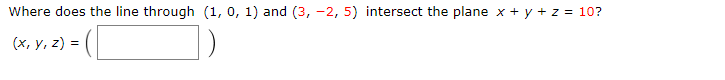Home / Answered Questions / Other / where-does-the-line-through-1-0-1-and-3-2-5-intersect-the-plane-x-y-z-10-x-y-z-aw679

# (Solved): Where Does The Line Through (1, 0, 1) And (3, -2,5) Intersect The Plane X + Y + Z = 10? (x, Y, Z) =(...Where does the line through (1, 0, 1) and (3, -2,5) intersect the plane x + y + z = 10? (x, y, z) =(

We have an Answer from Expert